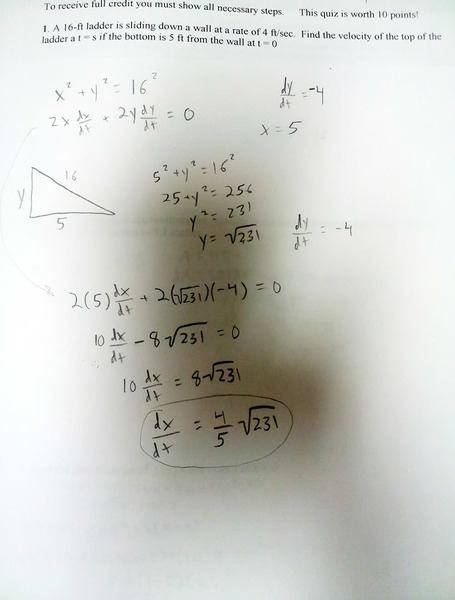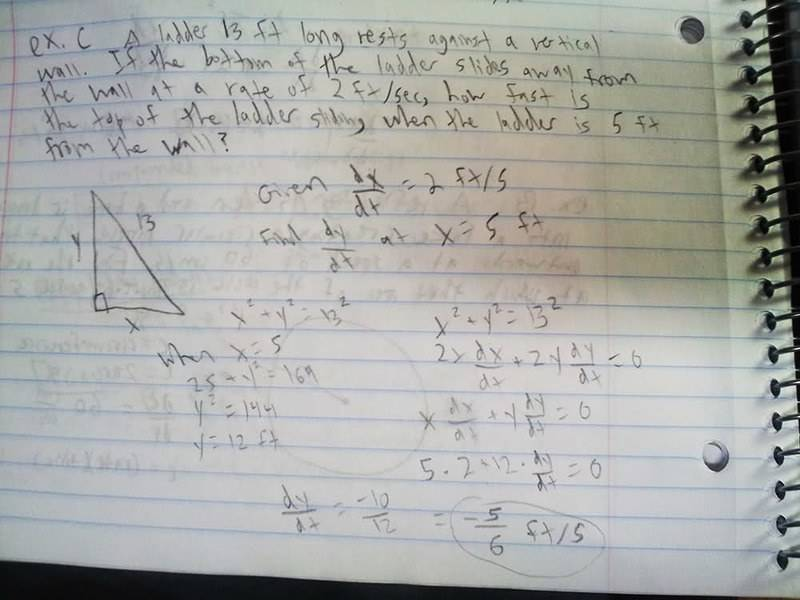# Help with related rates problem

• bl4ke360

## Homework Statement

A 16-ft ladder is sliding down a wall at a rate of 4 ft/sec. Find the velocity of the top of the ladder at t=s if the bottom is 5ft from the wall at t=0.

## Homework Equations

It's a related rates problem.
Not sure how I'm supposed to incorporate t=s into the problem, or if I'm supposed to solve for dx/dt or dy/dt.
Or if I'm even on the right track.

## The Attempt at a SolutionLast edited:
Thought I would add, this is a similar problem that was in my notes, but there are differences between the two problems such as the direction the rate of the ladder is sliding and I have to find it at t=s.This is due in a few hours I hope I get a response by then.

Just turned it in, thanks for the help everybody :)